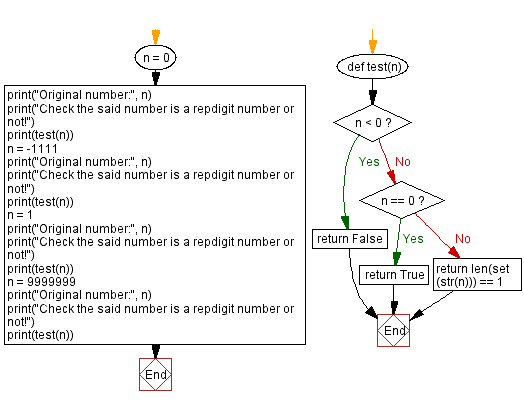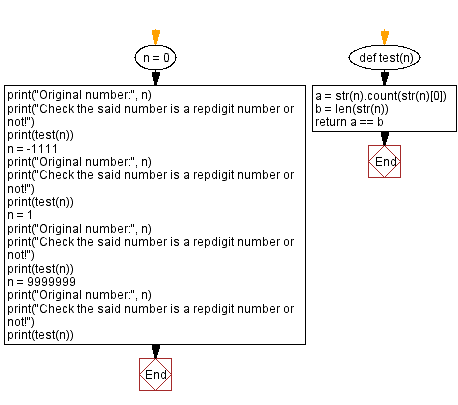﻿ Python - Check a number is a repdigit number or not

# Python Exercises: Check a number is a repdigit number or not

## Python Math: Exercise-89 with Solution

From Wikipedia,
In recreational mathematics, a repdigit or sometimes monodigit is a natural number composed of repeated instances of the same digit in a positional number system. The word is a portmanteau of repeated and digit. Examples are 11, 666, 4444, and 999999.

Write a Python program to check if a given number is a repdigit number or not. If the given number is repdigit return true otherwise false.

Sample Data:
(0) -> True
(1) -> True
(-1111) -> False
(9999999) -> True

Sample Solution-1:

Python Code:

``````def test(n):
if n < 0: return False
if n == 0: return True
return len(set(str(n))) == 1
n = 0
print("Original number:", n)
print("Check the said number is a repdigit number or not!")
print(test(n))
n = -1111
print("Original number:", n)
print("Check the said number is a repdigit number or not!")
print(test(n))
n = 1
print("Original number:", n)
print("Check the said number is a repdigit number or not!")
print(test(n))
n = 9999999
print("Original number:", n)
print("Check the said number is a repdigit number or not!")
print(test(n))
```
```

Sample Output:

```Original number: 0
Check the said number is a repdigit number or not!
True
Original number: -1111
Check the said number is a repdigit number or not!
False
Original number: 1
Check the said number is a repdigit number or not!
True
Original number: 9999999
Check the said number is a repdigit number or not!
True
```

Flowchart:## Visualize Python code execution:

The following tool visualize what the computer is doing step-by-step as it executes the said program:

Sample Solution-2:

Python Code:

``````def test(n):
a = str(n).count(str(n))
b = len(str(n))
return a == b

n = 0
print("Original number:", n)
print("Check the said number is a repdigit number or not!")
print(test(n))
n = -1111
print("Original number:", n)
print("Check the said number is a repdigit number or not!")
print(test(n))
n = 1
print("Original number:", n)
print("Check the said number is a repdigit number or not!")
print(test(n))
n = 9999999
print("Original number:", n)
print("Check the said number is a repdigit number or not!")
print(test(n))
```
```

Sample Output:

```Original number: 0
Check the said number is a repdigit number or not!
True
Original number: -1111
Check the said number is a repdigit number or not!
False
Original number: 1
Check the said number is a repdigit number or not!
True
Original number: 9999999
Check the said number is a repdigit number or not!
True
```

Flowchart:## Visualize Python code execution:

The following tool visualize what the computer is doing step-by-step as it executes the said program:

Python Code Editor:

Have another way to solve this solution? Contribute your code (and comments) through Disqus.

Previous Python Exercise: Check whether a given number is a Disarium number or unhappy number.
Next Python Exercise: Check if a number is a Harshad number or not.

What is the difficulty level of this exercise?

Test your Programming skills with w3resource's quiz.

﻿

## Python: Tips of the Day

Get Current Process Id:

```import os
os.getpid()
```
`21423`

We are closing our Disqus commenting system for some maintenanace issues. You may write to us at reach[at]yahoo[dot]com or visit us at Facebook